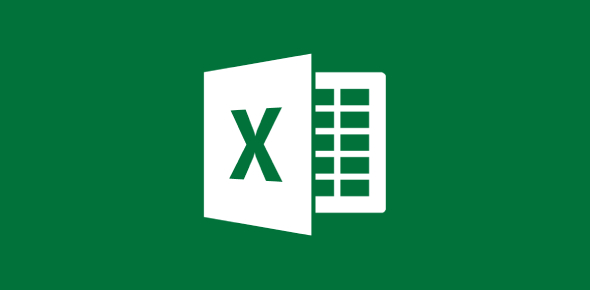# Microsoft Excel Quiz: Can You Answer?

30 Questions | Attempts: 325
ShareSettings.

• 1.
When you open up Microsoft Excel, you open it up to a _______________.
• A.

• B.

Worksheet

• C.

Sheet

• 2.
A row is __________.
• A.

Horizontal

• B.

Vertical

• 3.
A column is ____________.
• A.

Horizontal

• B.

Vertical

• 4.
To merge two are more cells together use the ________________.
• A.

Tab key

• B.

Insert

• C.

Merge & center

• D.

Copy & paste

• 5.
What command do you use if you want to save your excel assignment under a different name?
• A.

Save

• B.

Save as

• C.

Options

• D.

Save up

• 6.
When putting a formula in, what symbol must you put in first?
• A.

+

• B.

#

• C.

>

• D.

=

• 7.
If subtracting two items from each other, what word command must you place after the symbol.
• A.

SUM

• B.

SUB

• C.

MIN

• D.

• 8.
What is the name of the bar where you put in a formula?
• A.

• B.

Formula bar

• C.

Ribbon bar

• D.

Symbol bar

• 9.
When the formula contains the address of a cell, it is called a(n):
• A.

• B.

Group

• C.

Cell reference

• D.

Order of operations

• 10.
Worksheets can be:
• A.

Named

• B.

• C.

Duplicated

• D.

All of the above

• 11.
If you are printing paper the long way, you the orientation is:
• A.

Regular

• B.

Vertical

• C.

Portrait

• D.

Landscape

• 12.
When copying information from one cell to another, and the cell information stays the same, that is called:
• A.

Copy cells

• B.

Fill formatting

• C.

Fill without formatting

• D.

Copy and paste

• 13.
To create a chart or graph you must use the tab marked:
• A.

Home

• B.

View

• C.

Formula

• D.

Insert

• 14.
To sort in descending order means to sort:
• A.

A - z

• B.

Z - a

• 15.
A pie chart is used to:
• A.

Show information and date over a period of time

• B.

Create a pretty graph with different types of colors

• C.

Show information in relationship to each other

• 16.
A column chart :
• A.

Goes up and down

• B.

Goes side to side

• C.

Goes in circles

• D.

Goes in a line

• 17.
A cell is identified by two characters, often referred to as:
• A.

Numericalpha

• B.

Lettersnumbers

• C.

Numbersletters

• D.

Alphanumeric

• 18.
When heading information is placed in cell 1 (one) of each column, what is it called?
• A.

• B.

• C.

• D.

• 19.
What can excel be used for?
• A.

• B.

Create phone book

• C.

Cost of vacation

• D.

Create labels

• E.

Some of the above

• F.

All of the above

• 20.
If you were to add two numbers and multiply them by 15, what would the equation look like in excel?
• A.

=a1 + b1 x 15

• B.

=sum(a1 + b1)x15

• C.

=sum(a1 + b1)*15

• D.

Sum(a1 + b1) /15

• 21.
• A.

True

• B.

False

• 22.
Excel is a program.
• A.

True

• B.

False

• 23.
Excel can be used to create a database.
• A.

True

• B.

False

• 24.
At Central High School we use MS Excel 10.
• A.

True

• B.

False

• 25.
When making a graph, you have to highlight everything.
• A.

True

• B.

False

## Related TopicsBack to top
×

Wait!
Here's an interesting quiz for you.